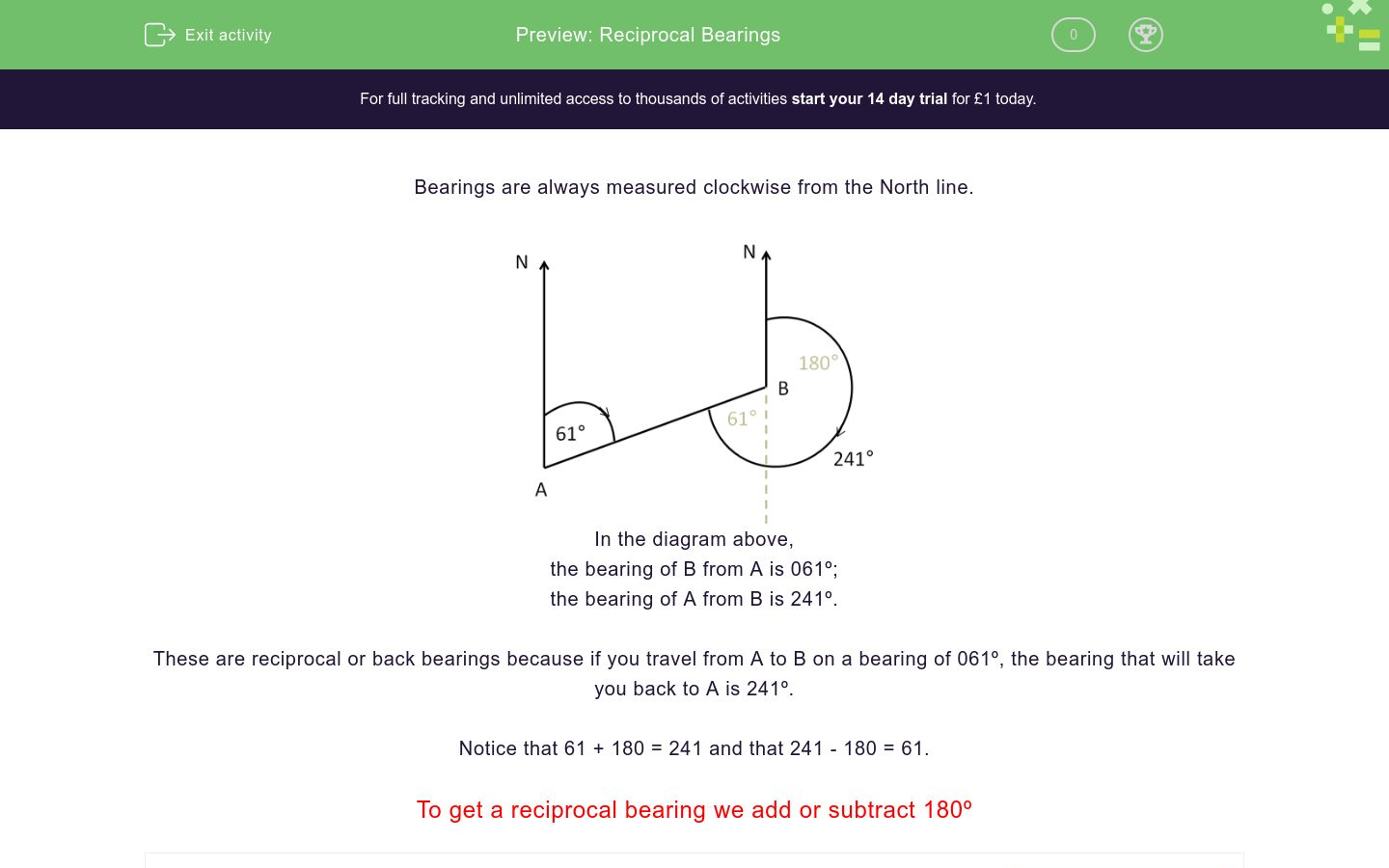# Reciprocal Bearings

In this worksheet, students calculate reciprocal bearings.Key stage:  KS 4

Curriculum topic:  Geometry and Measures

Curriculum subtopic:  Interpret and Use Bearings

Difficulty level:### QUESTION 1 of 10

Bearings are always measured clockwise from the North line.In the diagram above,

the bearing of B from A is 061º;

the bearing of A from B is 241º.

These are reciprocal or back bearings because if you travel from A to B on a bearing of 061º, the bearing that will take you back to A is 241º.

Notice that 61 + 180 = 241 and that 241 - 180 = 61.

To get a reciprocal bearing we add or subtract 180º

The bearing of B from A is 050º.

Calculate the reciprocal bearing (i.e. A from B)

(just write the three-figure number)

The bearing of B from A is 085º.

Calculate the reciprocal bearing (i.e. A from B)

(just write the three-figure number)

The bearing of B from A is 135º.

Calculate the reciprocal bearing (i.e. A from B)

(just write the three-figure number)

The bearing of B from A is 315º.

Calculate the reciprocal bearing (i.e. A from B)

(just write the three-figure number)

The bearing of B from A is 343º.

Calculate the reciprocal bearing (i.e. A from B)

(just write the three-figure number)

The bearing of B from A is 007º.

Calculate the reciprocal bearing (i.e. A from B)

(just write the three-figure number)

The bearing of B from A is 164º.

Calculate the reciprocal bearing (i.e. A from B)

(just write the three-figure number)

The bearing of B from A is 181º.

Calculate the reciprocal bearing (i.e. A from B)

(just write the three-figure number)

The bearing of B from A is 200º.

Calculate the reciprocal bearing (i.e. A from B)

(just write the three-figure number)

The bearing of B from A is 000º.

Calculate the reciprocal bearing (i.e. A from B)

(just write the three-figure number)

• Question 1

The bearing of B from A is 050º.

Calculate the reciprocal bearing (i.e. A from B)

(just write the three-figure number)

230
EDDIE SAYS
50 + 180
• Question 2

The bearing of B from A is 085º.

Calculate the reciprocal bearing (i.e. A from B)

(just write the three-figure number)

265
EDDIE SAYS
85 + 180
• Question 3

The bearing of B from A is 135º.

Calculate the reciprocal bearing (i.e. A from B)

(just write the three-figure number)

315
EDDIE SAYS
135 + 180
• Question 4

The bearing of B from A is 315º.

Calculate the reciprocal bearing (i.e. A from B)

(just write the three-figure number)

135
EDDIE SAYS
315 - 180
• Question 5

The bearing of B from A is 343º.

Calculate the reciprocal bearing (i.e. A from B)

(just write the three-figure number)

163
EDDIE SAYS
343 - 180
• Question 6

The bearing of B from A is 007º.

Calculate the reciprocal bearing (i.e. A from B)

(just write the three-figure number)

187
EDDIE SAYS
7 + 180
• Question 7

The bearing of B from A is 164º.

Calculate the reciprocal bearing (i.e. A from B)

(just write the three-figure number)

344
EDDIE SAYS
164 + 180
• Question 8

The bearing of B from A is 181º.

Calculate the reciprocal bearing (i.e. A from B)

(just write the three-figure number)

001
EDDIE SAYS
181 - 180
• Question 9

The bearing of B from A is 200º.

Calculate the reciprocal bearing (i.e. A from B)

(just write the three-figure number)

020
EDDIE SAYS
200 - 180
• Question 10

The bearing of B from A is 000º.

Calculate the reciprocal bearing (i.e. A from B)

(just write the three-figure number)

180
EDDIE SAYS
0 + 180
---- OR ----

Sign up for a £1 trial so you can track and measure your child's progress on this activity.

### What is EdPlace?

We're your National Curriculum aligned online education content provider helping each child succeed in English, maths and science from year 1 to GCSE. With an EdPlace account you’ll be able to track and measure progress, helping each child achieve their best. We build confidence and attainment by personalising each child’s learning at a level that suits them.